# Math in Focus Grade 1 Chapter 12 Practice 1 Answer Key Counting to 40

Practice the problems of Math in Focus Grade 1 Workbook Answer Key Chapter 12 Practice 1 Counting to 40 to score better marks in the exam.

## Math in Focus Grade 1 Chapter 12 Practice 1 Answer Key Counting to 40

First make tens, then count on. Fill in the missing numbers.

Example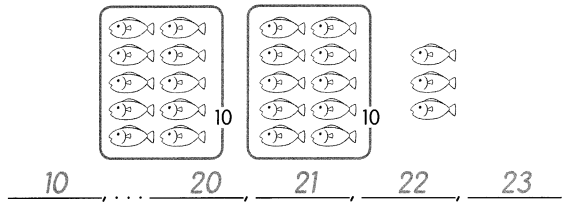Question 1.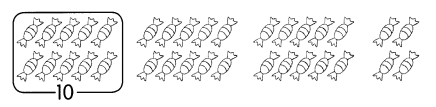____, . . . 20, . . . _____, 31, ____, ______
_______
Answer: 10, . . . 20, . . . 30, 31, 32, 33,34

Question 2.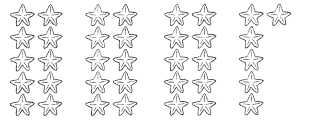10, . . . ____, . . . ____, ____, _____
33, ____, _____, 36
Answer:10, . . .20, . . . 30,31, 32,33, 34, 35, 36

Circle groups of 10. Then count and write the numbers.

Question 3.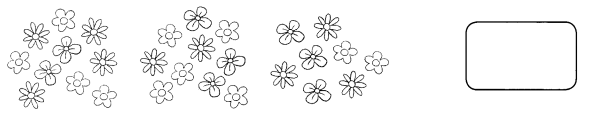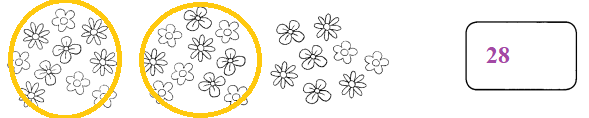Question 4.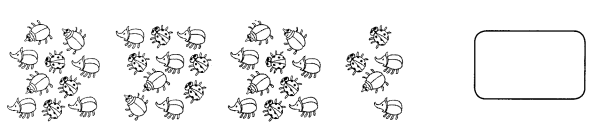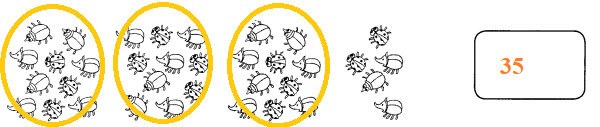Question 5.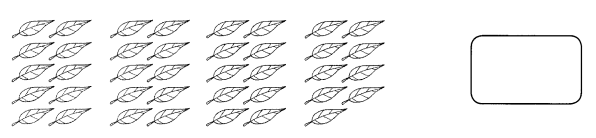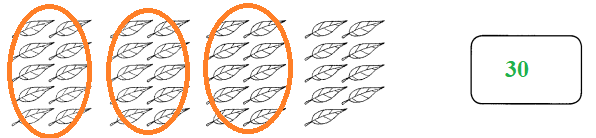Question 6.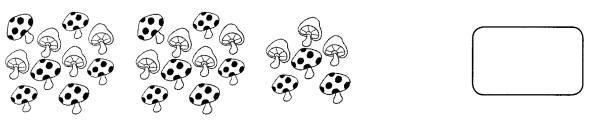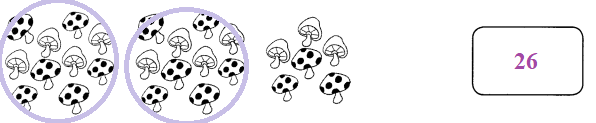Question 7.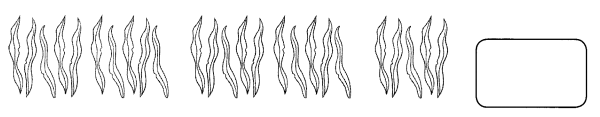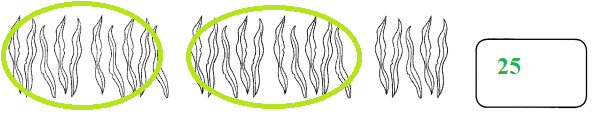Count the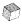s and write the numbers.

Question 8.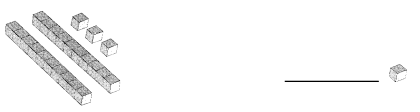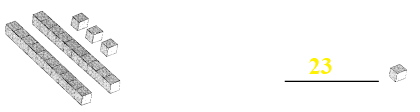Question 9.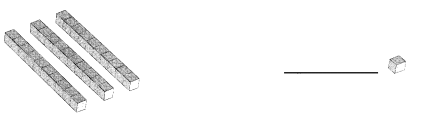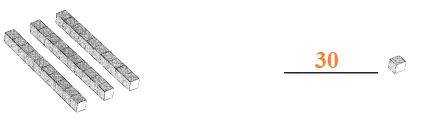Question 10.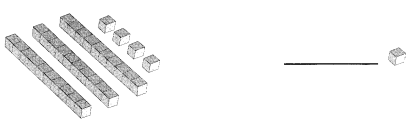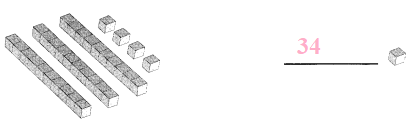Question 11.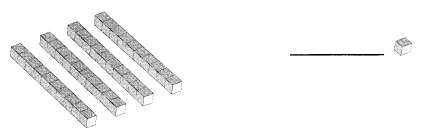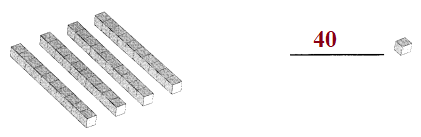Write the numbers.

Question 12.
twenty-five _______
Answer: twenty-five in numeric is 25

Question 13.
thirty-nine ____
Answer: thirty-nine in numeric is 39

Question 14.
thirty-two ____
Answer: thirty-two in numeric is 32

Question 15.
twenty-nine ____
Answer: twenty-nine in numeric is 29

Write the numbers in words.

Question 16.
21 _______________
Answer: 21 in words is twenty one

Question 17.
37 ________________
Answer: 37 in words is thirty seven

Question 18.
22 ____________
Answer: 22 in words is twenty two

Question 19.
40 _______________

Question 20.
35 _______________
Answer: 35 in words is thirty five

Question 21.
31 _______________
Answer: 31 in words is thirty one

Fill in the missing numbers.

Question 22.
20 + 3 = ____
Answer: 20 + 3 = 23

Question 23.
8 + 30 = ____
Answer: 8 + 30 = 38

Question 24.
_____ + 9 = 39
Answer: 30 + 9 = 39

Question 25.
30 and 5 make ___.
Answer: 30 and 5 make 35

Question 26.
7 and 20 make ___.
Answer: 7 and 20 make 27

Question 27.
___ and 2 make 32.
Answer: 30 and 2 make 32.

Question 28.
___ and 8 make 28.
Answer: 20 and 8 make 28.

Question 29.
___ and 6 make 36.
Answer: 30 and 6 make 36.